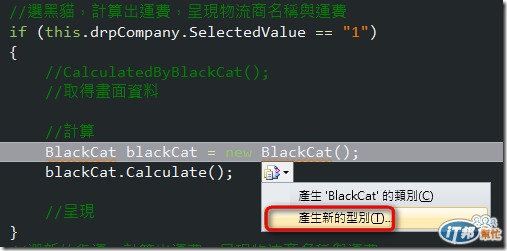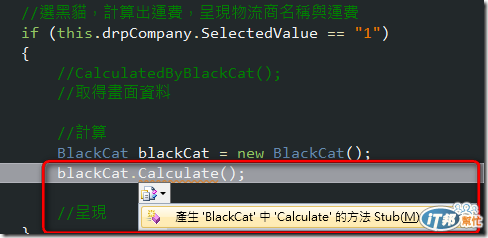#DAY 12
3

## [Day 12]Refactoring - 職責分離

＠目前的程式碼

``````protected void btnCalculate_Click(object sender, EventArgs e)
{
//若頁面通過驗證
if (this.IsValid)
{
//選黑貓，計算出運費，呈現物流商名稱與運費
if (this.drpCompany.SelectedValue == "1")
{
CalculatedByBlackCat();
}
//選新竹貨運，計算出運費，呈現物流商名稱與運費
else if (this.drpCompany.SelectedValue == "2")
{
CalculatedByHsinchu();
}
//選郵局，計算出運費，呈現物流商名稱與運費
else if (this.drpCompany.SelectedValue == "3")
{
CalculatedByPostOffice();
}
//發生預期以外的狀況，呈現警告訊息，回首頁
else
{
this.ClientScript.RegisterStartupScript(this.GetType(), "back", js, true);
}
}
}

private void CalculatedByPostOffice()
{
this.lblCompany.Text = "郵局";

var weight = Convert.ToDouble(this.txtProductWeight.Text);
var feeByWeight = 80 + weight * 10;

var length = Convert.ToDouble(this.txtProductLength.Text);
var width = Convert.ToDouble(this.txtProductWidth.Text);
var height = Convert.ToDouble(this.txtProductHeight.Text);
var size = length * width * height;
var feeBySize = size * 0.0000353 * 1100;

if (feeByWeight < feeBySize)
{
this.lblCharge.Text = feeByWeight.ToString();
}
else
{
this.lblCharge.Text = feeBySize.ToString();
}
}

private void CalculatedByHsinchu()
{
this.lblCompany.Text = "新竹貨運";
var length = Convert.ToDouble(this.txtProductLength.Text);
var width = Convert.ToDouble(this.txtProductWidth.Text);
var height = Convert.ToDouble(this.txtProductHeight.Text);

var size = length * width * height;

//長 x 寬 x 高（公分）x 0.0000353
if (length > 100 || width > 100 || height > 100)
{
this.lblCharge.Text = (size * 0.0000353 * 1100 + 500).ToString();
}
else
{
this.lblCharge.Text = (size * 0.0000353 * 1200).ToString();
}
}

private void CalculatedByBlackCat()
{
this.lblCompany.Text = "黑貓";
var weight = Convert.ToDouble(this.txtProductWeight.Text);
if (weight > 20)
{
this.lblCharge.Text = "500";
}
else
{
var fee = 100 + weight * 10;
this.lblCharge.Text = fee.ToString();
}
}
``````

＠重構第四式：誰，做什麼事。1. 蒐集頁面資訊供計算運費。
2. 呈現所選物流商名稱，以及計算完的運費結果。

1. CalculatedByBlackCat(): 透過黑貓來計算
2. CalculatedByHsinchu(): 透過新竹貨運來計算
3. CalculatedByPostOffice(): 透過郵局來計算

1. 主詞：代表類別
2. 動詞：代表方法
3. 受詞：通常是方法參數
4. 形容詞：通常是呼叫物件行為後，物件產生的狀態變化

1. CalculatedByBlackCat()：黑貓，計算運費
2. CalculatedByHsinchu()：新竹貨運，計算運費
3. CalculatedByPostOffice()：郵局，計算運費

1. CalculatedByBlackCat()改成：

``````        BlackCat blackCat = new BlackCat();
blackCat.Calculate();
``````
2. CalculatedByHsinchu()改成：

``````        Hsinchu hsinchu = new Hsinchu();
hsinchu.Calculate();
``````

3.CalculatedByPostOffice()改成：

``````            PostOffice postOffice = new PostOffice();
postOffice.Calculate();
``````

``````protected void btnCalculate_Click(object sender, EventArgs e)
{
//若頁面通過驗證
if (this.IsValid)
{
//選黑貓，計算出運費，呈現物流商名稱與運費
if (this.drpCompany.SelectedValue == "1")
{
//CalculatedByBlackCat();
//取得畫面資料

//計算
BlackCat blackCat = new BlackCat();
blackCat.Calculate();

//呈現
}
//選新竹貨運，計算出運費，呈現物流商名稱與運費
else if (this.drpCompany.SelectedValue == "2")
{
//CalculatedByHsinchu();
//取得畫面資料

//計算
Hsinchu hsinchu = new Hsinchu();
hsinchu.Calculate();

//呈現
}
//選郵局，計算出運費，呈現物流商名稱與運費
else if (this.drpCompany.SelectedValue == "3")
{
//CalculatedByPostOffice();
//取得畫面資料

//計算
PostOffice postOffice = new PostOffice();
postOffice.Calculate();

//呈現
}
//發生預期以外的狀況，呈現警告訊息，回首頁
else
{
this.ClientScript.RegisterStartupScript(this.GetType(), "back", js, true);
}
}
}
````````````public class BlackCat
{
public void Calculate()
{
throw new NotImplementedException();
}

public Product ShipProduct { get; set; }

public string GetsComapanyName()
{
throw new NotImplementedException();
}

public double GetsFee()
{
throw new NotImplementedException();
}
}
``````

＠小結

1. 註解加上人話。
2. 人話變成function
3. 找出人話中的主詞與動詞
4. 主詞變成類別（也就是物件）
5. 動詞變成方法 （也就是行為）

30天快速上手TDD31

### 1 則留言

0

hatelove提到：pajace2001 iT邦研究生 1 級 ‧ 2012-10-20 02:26:56 檢舉